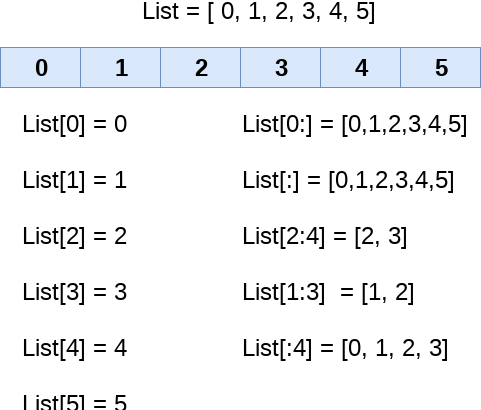# How to Sort List in Python

Sometimes you may need to sort list in python to sort in different ways. For example, you may need to sort a list of integers, floating point numbers, strings or other data types. In this article, we will look at how to sort list in Python. Python lists come with pre-built sort function that allows you to easily sort data in numerous ways.

## How to Sort List in Python

We will use the sort function to sort python lists in ascending, descending and user-defined order. Here is the syntax of sort function.

`list_name.sort([options])`

Also read : How to Install Google SDK in Ubuntu

## Sort list in ascending order

You can sort a python list in ascending order by simply calling the sort function on your list.

```>>> num_list=[4,2,1,3]
>>> num_list.sort()
>>> num_list
[1, 2, 3, 4]```

sort() function sorts both numbers and strings in ascending order. In case of strings, it will sort your list alphabetically.

```>>> str_list=['zebra','xray','yak','dove']
>>> str_list.sort()
>>> str_list
['dove', 'xray', 'yak', 'zebra']```

Also read : How to Configure UFW Firewall in Linux

## Sort list in descending order

You can sort python list in descending order by adding reverse=True option. Please note, you need to enter True with a capital T, else you will get an error.

```>>> num_list = [4,1,3,2]
>>> num_list.sort(reverse=True)
>>> num_list
[4, 3, 2, 1]```

Similarly, you can sort list of strings in descending order.

```>>> str_list=['zebra','xray','yak','dove']
>>> str_list.sort(reverse=True)
>>> str_list
['zebra', 'yak', 'xray', 'dove']```

Also read : How to Use NGINX with Flask

## Sort List with User-defined order

You can also sort python lists in user defined order. It is useful if your list consists of tuples or dictionary data types.

Here is the syntax to sort lists in a user-defined order.

`list_name.sort(key=...,reverse=...)`

Here is an example where sort a tuple based on its second element. We define a function get_second to get the second element of each tuple.

```>>> tuple_list = [(1, 2), (3, 3), (1, 1)]
>>> def get_second(val):
return val
>>> tuple_list.sort(key = get_second)
>>> tuple_list
[(1, 1), (1, 2), (3, 3)]```

Similarly, you can sort a list of tuples in descending order just by adding (reverse=True)

```>>> tuple_list.sort(key = get_second,reverse=True)
>>> tuple_list
[(3, 3), (1, 2), (1, 1)]```

In the above article, we have shown how to sort python list in ascending, descending and user-defined orders. Python lists are very powerful data structures that offer many functions in a very simple way.

Also read : How to Convert Integer to String in Python